# How to format Addresses in Excel

Here we have a dataset above which contains ten random addresses, we will be separating the addresses into multiple columns using some formulas. Step by step procedure is given below, let’s have a look at the dataset first.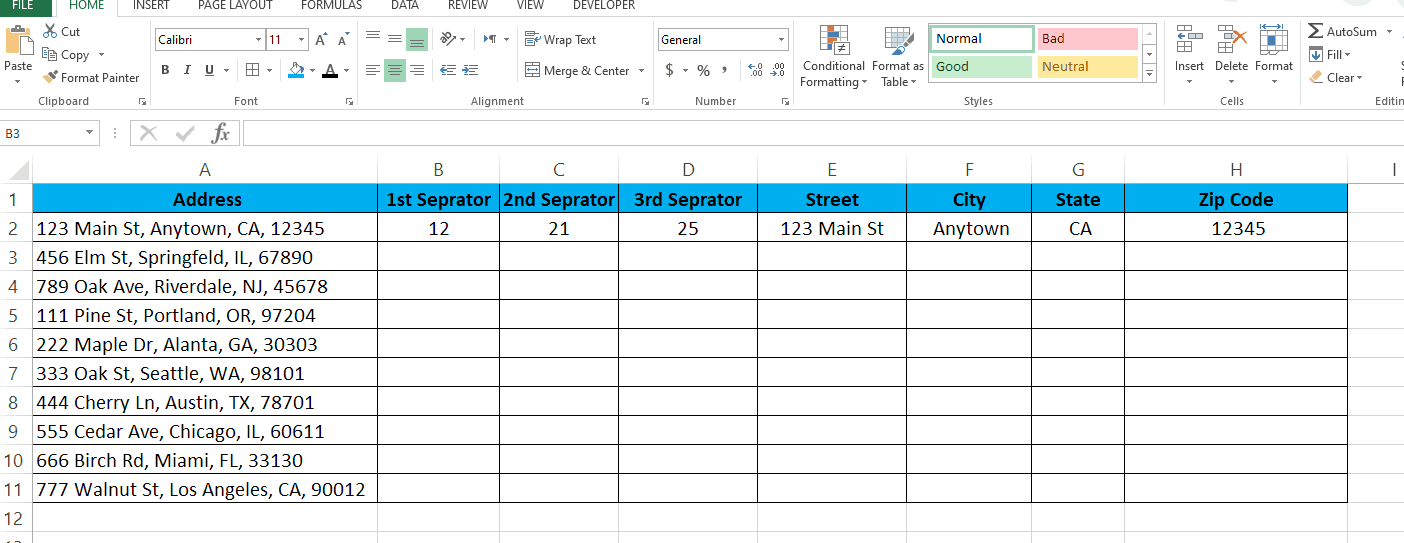When working with Excel, formatting addresses can be a bit tricky. However, with a few simple tips and tricks, you can easily format addresses in Excel. In this tutorial we will learn how to format addresses in Excel.

### Step 1 – Add the 1st Separator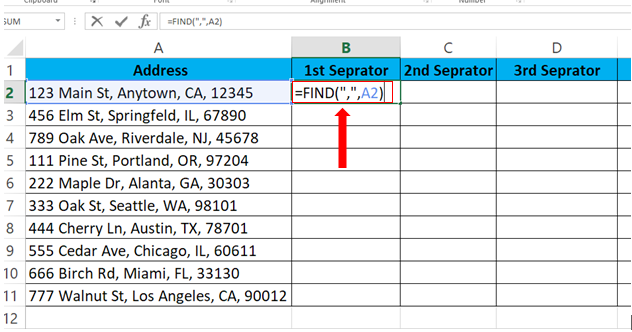– To add the first Separator, type in the formula.
– Syntax of the formula:
– In our case the formula will be
=FIND(“,”,A2)

### Step 2 – Add the 2nd Separator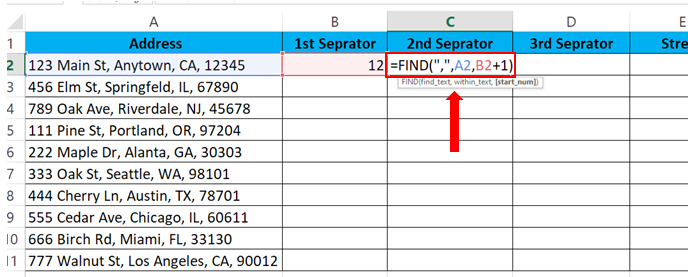– To add the second Separator, type in the formula.
– Syntax of the formula:
– In our case the formula will be
=FIND(“,”,A2,B2+1)

### Step 3 – Add the 3rd Separator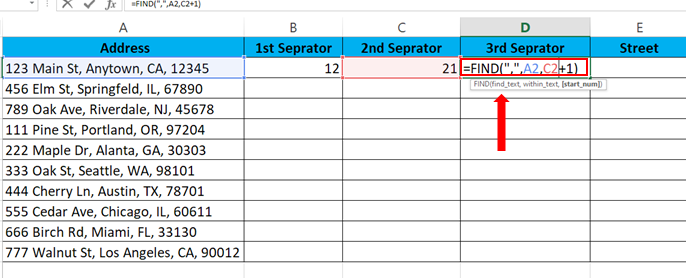– To add the third Separator, type in the formula.
– Syntax of the formula:
– In our case the formula will be
=FIND(“,”,A2,C2+1)

### Step 4 – Type the formula for the Street Address.– To find the Street type the formula in the cell
– Syntax of the formula is
– In our case the formula is
=LEFT(A2,B2-1)

### Step 5 – Type the formula for the City name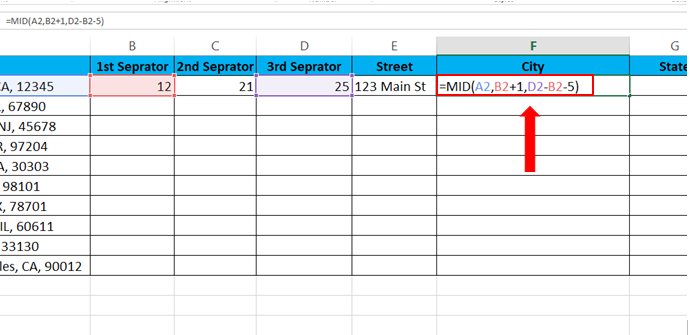To find the City type the formula in the cell
Syntax of the formula is
=MID(Cell_with_Address,1stSeparator + 1,3rdSeparator – 2ndSeparator – 5 )
In our case the formula is
=MID(A2,B2+1,D2-B2-5)

### Step 6 – Type the formula for the State name.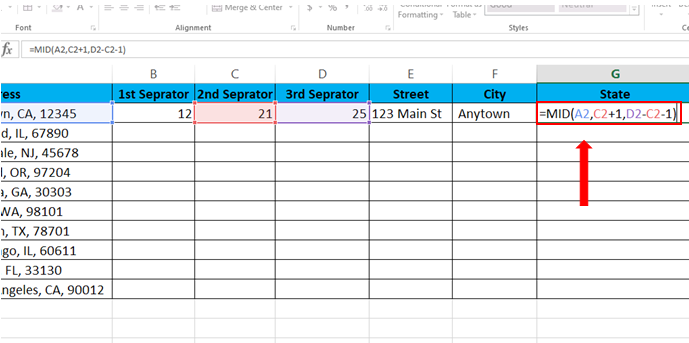– To find the State, type the formula in the cell
– Syntax of the formula is
=MID(Cell_with_Address,2nd_Separator + 1,3rd_Separator – 2nd_Separator – 1 )
– In our case the formula is
=MID(A2,C2+1,D2-C2-1)

### Step 7 – Type the formula for the Zip Code.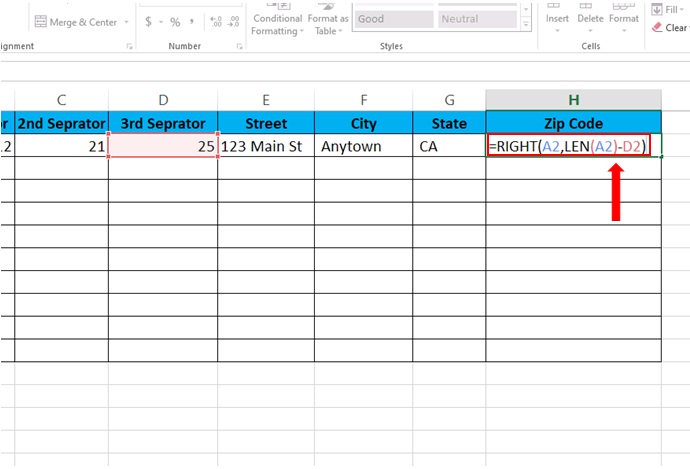– To find the Zip code, type the formula in the cell
– Syntax of the formula is